# Enhanced Heat Transfer by Oil/Multi-Walled Carbon Nano-Tubes Nanofluid

Enhanced Heat Transfer by Oil/Multi-Walled Carbon Nano-Tubes Nanofluid

Abdelhakim Boursas Mohamed Salmi Giulio LorenziniHijaz Ahmad Younes Menni Djamal Fridja

Department of Physics, University of M’sila, B.P. 1713, M’sila 28000, Algeria

Laboratory of Physics and Chemistry of Materials, University of M’sila, M’sila 28000, Algeria

Department of Engineering and Architecture, University of Parma, Parco Area delle Scienze, 181/A, Parma 43124, Italy

Department of Basic Science, University of Engineering and Technology, Peshawar 25000, Pakistan

Department of Physics, Faculty of Sciences, Abou Bekr Belkaid University, P.O. Box 119, Tlemcen 13000, Algeria

Corresponding Author Email:
Giulio.lorenzini@unipr.it
Page:
93-103
|
DOI:
https://doi.org/10.18280/acsm.450201
Received:
23 December 2020
|
Accepted:
12 March 2021
|
Published:
30 April 2021
| Citation

OPEN ACCESS

Abstract:

The subject of the study is mainly based on thermal reinforcement by an oily fluid containing nanometer particles of carbon. The study is carried out by the presence of discontinuous bars in two different shapes, i.e. flat and V, inside a horizontal heat exchanger. The study relies on simulations in thermal and dynamic terms from the literature. The turbulence effect is diagnosed by applying the k-ε model, while the flow hydrothermal transport relationships are modeled based on the finite volume technique. Both the flow and heat-transfer aspects of all channel regions are studied and analyzed. The new heat-exchanger structure has been enhanced in the presence of these discontinuous bars by reducing the friction coefficient and eliminating stations with poor transfer of heat behind these deflectors.

Keywords:

nanofluid, oil, multi-walled carbon nano-tubes, mechanical properties, thermodynamic properties

1. Introduction

In order to enhance heat-transfer and improve the performance of heat-exchangers, which are used in various industrial sectors, modern and more effective methods must be sought. Some researchers have shown interest in improving the physical structure of the heat-transfer fluid. Most of the studies are based on traditional fluids such as water, especially air. The results obtained show a slight thermal enhancement due to the decrease in the thermal-conductivity of such conventional fluids. Some other studies resorted to increasing thermal-conductivity by adding nanoscale solid particles capable of excessively absorbing thermal-energy from hot walls [1-3]. In such contributions, researchers found enhanced thermal-transport over channels under the presence of aerobic or aqueous fluids.

Different studies with distinct boundary conditions have been carried out in the presence of porous media in order to limit the high friction values . Most of these studies were performed for conventional fluid heat-exchangers, where a higher performance was recorded compared to the case without a porous medium. On the other hand, nanofluidic fluxes are modeled in the presence of the porous media . The effect of the thermal and mechanical properties of the fluid, as well as the characteristics of the porous medium, have been the subject of studies, which have shown the effectiveness of combining the two techniques together, i.e. using the nanofluid to activate the thermal-conductivity of the fluid, while embodying the porous medium inside the channel to reduce the charge loss, which enhances the effectiveness of the heat exchanger dynamically and energetically. From the engineering side of the exchanger, some studies have been established on the structure of the inner channel, meaning the use of rough surfaces instead of smooths surfaces, such as placing pins or adding extended spaces on the hot sides in order to extend the heat-exchange areas, or on the adiabatic walls for good fluid mixing [6, 7]. The incoming process improved both turbulence and convection phenomena, which is required. The majority of these contributions dealt with conventional fluids, highlighting several disadvantages, including the excessive rise in friction values, which negatively affected the heat transfer values and thus, reduced performance values.

The strategy of using the technique of stretched surfaces varied from one study to another. For example, vertical finned channels have been studied . While, researchers resorted to changing the angle of attack of these deflectors in both directions, the front and rear of the fluid field . The results were different for different inclination angle. The vertical turbulators showed higher coefficients of friction. Moreover, the inclined obstacles against the flow direction showed a large increase in the friction loss, in contrast to the behavior of the inclined baffles towards the outlet of the exchanger which showed a decrease in the pressure drop.

In order to reduce the height of the coefficient of friction, the authors modified the structure of the baffles by using porous materials  or by making holes  in order to permeate the fluid and reduce he pressure on the frons faces of these turbulators, thus reducing the charge loss.

Modeling the baffles in terms of their geometry is the goal and path of many researchers in order to update the thermal and energy performance of various solar exchangers (see Kamali and Binesh , Wang et al. , Fawaz et al. , Boonloi and Jedsadaratanachai , Tamna et al. , Nanan et al. , Menni [18-35] in Table 1). The effect of both deflector dimension and flow rate has also been analyzed for complex geometrical obstacles, such as helical baffles.

Table 1. Various numerical study on baffled channel heat exchangers

 Investigator (s) Physical domain Obstacle geometry Reynolds number (×103) Numerical method Numerical model Kamali and Binesh  Square ducts Quilateral-triangular, square, and Trapezoidal 8 to 20 Finite volume SST k-ω Wang et al.  Rectangular channel Drop 4.8 to 8.2 Finite volume Standard k-ε Fawaz et al.  Square channel 45° in-line 'V' 5 to 25 Finite volume RNG k-ε Boonloi and Jedsadaratanachai  Square channel Discrete combined 5 to 20 Finite volume Realizable k-ε Tamna et al.  Solar air heater channel Multiple 'V' 4 to 21 Finite volume RNG k-ε Nanan et al.  Heat exchanger tube Twisted 6 to 20 Finite volume Realizable k-ε Menni et al.  Rectangular channel Flat and 45° 'V' with vs. orientations 12 to 32 Finite volume- Standard k-ε Menni et al.  Rectangular channel 45° 'V' with vs. spacings 12 to 32 finite volume Standard k-ε Menni et al.  Solar air channels Simple, triangular, trapezoidal, cascaded, diamond, arc, corrugated, +, S, V, W, Z, T, Γ, and ε 12 to 32 Finite volume Standard k-ε Menni et al.  Channel heat exchangers Arc with vs. orientations 12 to 32 Finite volume Standard k-ε Menni et al.  Rectangular channel Detached and attached 5 to 50 Finite volume Standard k-ε Menni et al.  H2 fluid solar channels Detached and Attached 5 to 20 Finite volume Standard k-ε Menni et al.  Channel exchanger Vertical and 15°-75° inclined 5 to 20 Finite volume SST k-ω Menni et al.  Oil solar channels Staggered flat 5 to 25 Finite volume Standard k-ε Menni et al.  Solar duct Multiple 'V' 5 to 30 Finite volume Standard k-ε Menni et al.  Channel heat exchanger Cascaded, rectangular, triangular 12 to 32 Finite volume Standard k-ε Menni et al.  Rectangular channel Downstream 'V' 12 to 32 Finite volume Standard k-ε Menni et al.  Rectangular duct 'S'-upstream and 'S'-downstream 12 to 32 Finite volume Standard k-ε Menni et al.  Solar air channels Arc with vs. positions 12 Finite volume Standard k-ε Menni et al.  Solar air channels '+' 12 to 32 Finite volume Standard k-ε Menni et al.  Rectangular channel Waisted triangular 5 to 200 Finite volume Standard k-ε Menni et al.  Baffled channel Complex 12 to 32 Finite volume Standard k-ε Menni et al.  Solar air-heat exchanger 'ε' 17 to 32 Finite volume Standard k-ε Menni et al.  Horizontal duct Cascaded rectangular-triangular 10 to 30 Finite volume SST k-ω Menni et al.  2D channel 'L' 12 to 30 Finite volume SST k-ω Menni et al.  Rectangular channel Diamond 12 to 32 Finite volume Standard k-ε

Depending on previous studies, the reinforcement techniques can be summarized as follows: (i) the porous medium shows a decrease in pressure over the flow surfaces; (ii) the nanofluid shows an increase in the convective heat exchange coefficients and; (iii) the extended areas augment the friction values, which affects the heat transfer rates. According to these strategies, two techniques are used in this study simultaneously, namely, reinforcement of the disordered structure by gap bars of different shapes. These deflectors enhance the intensity of the turbulence, while the gaps reduce pressure and eliminate counter-flows with poor heat-transfer. This study is also carried out in the presence of a high thermal transfer fluid due to its physical properties. The two technologies will improve both the hydrodynamic and thermal aspects of the fluid flow field.

2. Physical Domain

2.1 Channel heat exchanger model

The study is carried out according to a two-dimensional simulation of a horizontal heat exchanger. The duct is an update of one of the simple baffled heat-air exchangers mentioned by Demartini et al. . This conduit has been studied by many researchers in order to augment its efficiency by giving a new structure to the simple baffles. For this purpose, Menni et al.  reported a new baffle type, represented by the V-shape. Their analysis of the results behind the baffles reported reverse flows with low thermal gradients, which affected the phenomenon of forced convection in such areas. Their interpretation showed two main reasons for this weakness in heat transfer, first, the decrease in the pressure on the back areas of the baffles, while the second is the high coefficient of friction in the gape, due to the presence of such extended surfaces.

Based on these analyzes, the motivation of this simulation suggests changing the simple baffles of the heat exchanger (Figure 1a). The study proposes two modern models.

(i) the first structure, is a flat and discontinuous baffle with gapes. In the upper part of the exchanger, two adjacent baffles are placed. One of them has three gaps (first upper flat baffle: FUFB), while the second baffle (second upper flat baffle: SUFB) contains only two gaps, in addition to the third gap between it and the top surface of the exchanger.

(ii) The second structure, is a V-baffle with an attack of 45° relative to the horizontal axis of the exchanger. Similarly, two adjacent baffles on the same underside with narrow gapes. On the right, the first formed baffle (first lower V-baffle: FLVB) has two gaps, while on the left, the second formed baffle (second lower V-baffle SLVB) has only one gap.

According to this model, the following are done: (i) the pressure values on the front areas of the baffles are reduced through the presence of holes, that is, a reduction in the values of fluid-solid friction, thus reducing the charge loss. This process greatly enhances the heat transfer of the exchanger; (ii) This structure allows the creation of two different flows, a main stream, that passes below the FUFB and SUFB, and above the FLVB and SLVB. While, a second flow exists in a secondary form where it crosses all the holes found in the FUFB, SUFB, FLVB and SLVB. The secondary fluid flow, through the holes, eliminates all back recirculation fields and, greatly enhance the exchanger transfer.

The structure adopted and proposed for the development of this model of exchangers helps to increase the transfer of heat across the conduit by reducing the increase in friction and eliminating the low heat transfer reverse flows, by secreting secondary flows through the baffle holes proposed by its new model in this study.

The originality of this simulation is the analysis of this new configuration of exchangers, in the presence of a discontinuous baffle structure, which allows the addition of secondary flows and elimination of recycling streams. This new dynamic behavior is a result of the new structure of this heat exchanger.

## 1a.png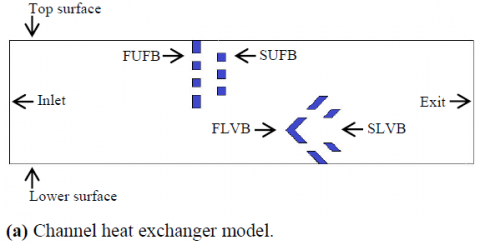## 1b.png## 1c.pngFigure 1. Simulated physical model

2.2 Geometrical parameters

The geometric dimensions tested in this simulation have been adopted in the literature for heat exchangers established with simple deflectors . In the second half of this same figure (Figure 1b), all the geometrical dimensions of the exchanger are shown. The FUFB and FLVB deflectors have the same height, estimated at 8 cm while, the other turbulators (SUFB and SLVB) are shorter, estimated at 5 cm. All holes received for FUFB and SUFB are square shape, side length is 1 cm. Whereas, the holes contained on the lower deflectors (FLVB and SLVB) are of parallelogram shape with the same dimension, i.e. 1 cm. The distance between each two adjacent baffles is 2 cm in both cases (FB and VB). Further, the FUFB is located 21.8 cm from the exchanger entrance, 32.6 cm from the exit, while 14.2 com from the FLVB. Finally, as for the dimensions of exchanger conduit, it is 55.4 cm for the length while 14.6 cm for the width. The thickness of the baffles is estimated at 1 cm, while it is neglected in the case of the exchanger surfaces. Both the surfaces of the exchanger and the areas of the baffles physically are the Aluminum. This model of the exchanger remains open for many future studies in order to develop it geometrically as well as in terms of its physical structure. It can also be treated by simulating in unsteady three-dimensions and experimentally with the effect of solar radiations instead of the temperature factor.

2.3 Limit conditions

Figure 1c highlights the characteristics of all surfaces of the exchanger. The inlet of the exchanger conduit is subjected to a fluid flow with an axial speed related to the Re values (Reynolds number), as stated in some indexed papers such as Menni et al. [18-35], Demartini et al. , and Nasiruddin and Kamran Siddiqui . Five different speeds are simulated in order to demonstrate the effect of flow rate on various dynamic and thermal phenomena. Dynamically, velocity changes and coefficients of friction can be studied while thermally, the temperature fields and developments of heat transfer values can be analyzed.

On the other hand, the outlet of the exchanger is simulated in terms of pressure. In this section, the fluid pressure is equal to the atmospheric pressure, as it has been discussed in many research studies such as Menni et al. [18-35], and Demartini et al. .

The study is also carried out under the influence of constant temperature of 300 K  for the entire upper wall of the exchanger opposite to the lower, insulated wall.

3. Numerical Model

3.1 Oil/multi-walled carbon nano-tubes (Oil/MWCNT)

The study is based on a new fluid type, which is the nanofluid. This simulation is an update of conventional aqueous fluids (water, oil, etc.) that have low dynamic and thermal behavior, due to their low physical properties. The motivation for this study lies in the search for the best fluids that transfer the heat. This requires an improvement at the nanometric level, such as adding solid particles (Al2O3, MWCNT, etc.) at low concentrations (≤ 6%) to the base fluid in order to create a new structure with improved properties, which allows the absorption of the largest possible part of the thermal energy by heat transfer from the hot spaces. The working fluid simulated is an oily liquid with carbon-type nanoscale solid particles at a fraction of 2% (Oil/MWCNT nanofluid). The physical properties of oil, MWCNT, and Oil/MWCNT are shown in Derakhshan et al.  and Gholami et al. .

3.2 Flow model

This simulation is don according to the following:

• The Oil/MWCNT flow is 2D;
• The Oil/MWCNT flow is turbulent;
• The Oil/MWCNT flow is incompressible;
• The Oil/MWCNT flow is Newtonian;
• The Oil/MWCNT flow is turbulent;
• The Oil/MWCNT flow is steady;
• The Oil/MWCNT flow is single phase;
• The working fluid (Oil/MWCNT) temperature is 298 K.
• The thermal transfer is done by the forced convection, while the transfer by radiation is canceled.
• The upper and lower surfaces of the exchanger have a negligible thickness.
• For all solid, a non-slip condition has been applied.
• The flow rate (Re × 103) is 12 to 32.
• The disturbance modeling model is k-ε model . Similar simulations from the literature are done under the same model as Wang et al. , Demartini et al. , Yang and Hwang , Biçer et al. , Lv et al. , etc.

3.3 Governing equations

For the abovementioned assumptions, the governing equations are written as:

Continuity:

$\frac{\partial}{\partial x_{i}}\left(\rho u_{i}\right)=0$   (1)

Momentum:

$\frac{\partial}{\partial x_{i}}\left(\rho u_{i} u_{j}\right)=\frac{\partial}{\rho x_{i}}\left[\mu\left(\frac{\partial u_{i}}{\partial x_{j}}-\rho \overline{u_{i}^{\prime} u_{j}^{\prime}}\right)\right]-\frac{\partial P}{\partial x_{i}}$  (2)

where, P, ρ, and μ are the pressure, fluid density, dynamic viscosity. ui and uj are mean velocity components in xi and xj directions.

Energy:

$\frac{\partial}{\partial x_{i}}\left(\rho u_{i} T\right)=\frac{\partial}{\rho x_{i}}\left(\left(\Gamma+\Gamma_{t}\right) \frac{\partial T}{\partial x_{j}}\right)$  (3)

where, Γt and Γ are, respectively, the turbulent thermal diffusivity and molecular thermal diffusivity, defined as:

$\Gamma=\mu / \mathrm{Pr}$ and $\Gamma_{t}=\mu_{t} / \mathrm{Pr}_{t}$  (4)

In Eq. (2), $\rho \overline{u_{i}^{\prime} u_{j}^{\prime}}$ is the Reynolds stresses defined by the Boussinesq hypothesis as:

$-\rho \overline{u_{i}^{\prime} u_{j}^{\prime}}=\mu_{t}\left(\frac{\partial u_{i}}{x_{j}}+\frac{u_{j}}{x_{i}}\right)-\frac{2}{3}\left(\rho k+\mu_{t} \frac{\partial u_{i}}{\partial x_{j}}\right) \delta_{i j}$  (5)

where, δij is the Kroenecker delta and μt the eddy viscosity defined as:

$\mu_{t}=\rho C_{\mu} \frac{k^{2}}{\varepsilon}$  (6)

The standard k-ε model is used for turbulence modeling. For further details on this model, the reader can refer to our previous paper . The Reynolds number (Re) is defined as:

$\operatorname{Re}=\rho \bar{U} D_{h} / \mu$  (7)

where, Dh is the hydraulic diameter, given by:

$D_{h}=2 H W /(H+W)$  (8)

The friction factor (f) is:

$f=\frac{(\Delta P / L) D_{h}}{\frac{1}{2} \rho \bar{U}^{2}}$  (9)

The local (Nux) and average (Nu) Nusselt numbers are, respectively, defined as:

$N u_{x}=\frac{h_{x} D_{h}}{k_{f}}$   (10)

where, $\bar{U}$ is the average axial velocity, and τw is the shear stress. The local (Nux) and average (Nu) Nusselt numbers are, respectively, defined as:

$N u=\frac{1}{L} \int N u_{x} \partial x$   (11)

All properties of the simulated nanofluid can be calculated using the relationships given in the references [9, 44].

3.4 Numerical approaches

The finite volume method is applied to solve the governing equations with the computer tool FLUENT. The convective and pressure terms are discretized with the QUICK and Second-order upwind schemes, respectively (Patankar ; Leonard and Mokhtari ). The pressure velocity coupling is done with the SIMPLE algorithm.

The under-relaxation factor that allows controlling the update of the predicted results at each step was changed in the range 0.3 - 1.0 (Nasiruddin and Kamran Siddiqui ). The results were considered converged when the residual target drop below 10-9 for pressure and velocities and 10-12 for the temperature. The computer tool GAMBIT is used for creating and meshing the computational domain.

4. Result and Discussion

To control the flow changes near the wall, the mesh is refined near the walls of the channel and baffles. Mesh dependency tests were carried out for several quadrilateral grid elements and the results reveal that the mesh with 245 × 95 (in X and Y coordinates, respectively) does not allow more variation than 0.350% in the values of Nusselt number. Therefore, this case was selected to perform all other calculations.

## 2.png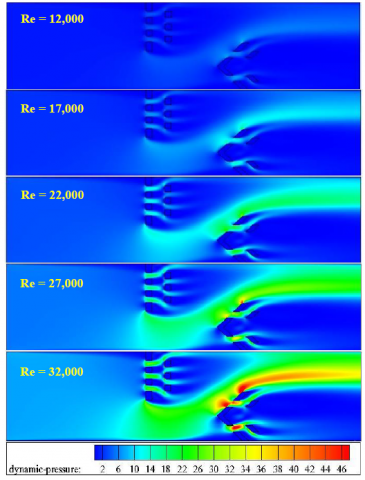Figure 2. Pd = f (Re)

4.1 Pd fields

Figure 2 shows the Pd (dynamic-pressure) evolution in terms of the Re. For a smaller value of the flow rate that is, for the minimum velocity at the inlet, there is a pressure drop over the entire exchanger structure. With the flow rate shifting from 12 to 32 (×103), the Pd value increases slightly on the front sides of both FUFB and FLVB. For an average value of the flow rate, the Pd value is slightly augmented through the holes of the four deflectors. When the flow rate is increased to 27 (×103), there is an enhancement in the pressure value below the FUFB and SUFB, and above the FLVB and SLVB. By maximizing the flow rate to its highest value, areas of high pressure appear through the two holes in the FLVB. Therefore, the pressure changes with the evolution of the flow rate from 12 to 32 (×103), being 45 Pa at Re = 12 (×103).

4.2 Ψ fields

The fluid has a constant velocity at the inlet of the conduit, and flows through the internal structure of the exchanger along lines. The field has a parallel configuration at this intake due to the fluid flowing at an axial speed. These lines are slightly tense in front of the FUFB. In the vicinity of the FUFB and SUFB, the fluid branches into two distinct streams.

## 3.png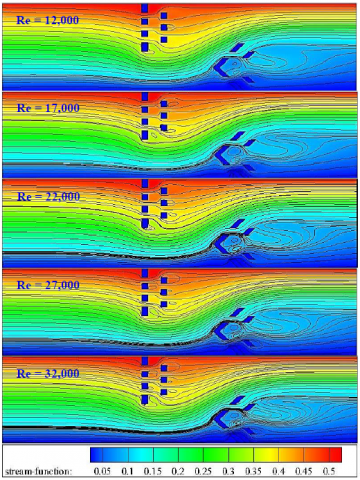Figure 3. Ψ = f (Re)

Secondary streams flow through the holes of both adjacent FUFB and SUFB. While, there is a main flied below these upper deflectors, it also flows in parallel lines until it reaches the FLVB and SLVB (Figure 3). The same dynamic behavior occurs in this region where the main stream splits into secondary fields through the holes, and the main field through the gaps. The role of the secondary flows is to eliminate all the recycling zones behind all the FBs and VBs, so this effectively reflects the presence of the discontinuous deflectors. Also, from the plot, the flow lines are greatly strained with the continuous increase in flow rates.

4.3 V fields

The speed-magnitude (V) has different values from one zone to another (Figure 4). The V value is low in the front space of the exchanger, from x/L = 0 to x/L = 0.393, due to the positioning of the upper expanses (FUFB and SUFB). It is also lowered downstream of the solid surfaces of these same deflectors as the Pd on the right sides of both FUFB and SUFB decreases. On the other hand, it is low extensively behind the upper second FB and to the right of the lower 2nd VB. The V value increases in four main locations of the exchanger. Secondary flows through holes have high speeds, due to high Pd over the entire frontal area of these deflectors in both cases simulated. The main currents that traverse the two gaps, the lower, i.e. below the FUFB and SUFB, and the upper, i.e. above the FLVB and SLVB, increase in speed, due to the increased Pd in the region. Significant progression of the flow speed is also evident over the entire path near the upper FLVB wing. The V value in the case of the lowest rate of flow is estimated at 3.18 m.s-1, i.e. an average value of 0.95 m.s-1. These values improve to 8.73 m.s-1 as the maximum velocity while to 2.62 m.s-1 as the medium value in the case of the highest rate of flow. The effect of Re, is reflected by the strong change in V from one state to another.

## 4.pngFigure 4. V = f (Re)

4.4 X-velocity fields

Figure 5 shows negative u values (x-velocity) which are most clearly evident in several aspects of the exchanger. Very limited areas lie behind the solid parts of both upper FBs also, to the left of the front head of the FLVB, as well as downstream of the solid parts of the SLVB adjacent to it. These areas contain weak vortices that are created due to low Pd values.

These vortices usually arise behind each low pressure surface, i.e. to the left of all continuous deflectors. All engineering properties of such phenomena mainly relate to the deflector dimensions. The most important influencing factors are the length of these vortex generators, their angle of attack and the distance between them. The size and strength of these rings change with the change in deflector geometry. Their strength increases with the length of the deflectors and the reduction in the separation between them. In this study, the presence of discontinuous FB and VB creates secondary flows that prevent the formation of vortices except for the small ones located behind the solid parts that make up these deflectors.

As for the main flow, its u is most valuable through the holes, especially those within the FLVB. The u also reaches large values in the last side of the exchanger, at its upper part, reaching 2.72 m.s-1 for the lowest flow rate, increasing by 43% with an increase in the Re to 17 (×103), 86% in the case of the next rate (Re = 22 × 103), 129 % in the case of Re = 27 (×103), while 172% in the case of the Re maximum (Re = 32 × 103). These speed improvements can also record as follows: 259%, 370%, 476%, 594% and 705% are greater than the inlet speed with Re = 12, 17, 22, 27 and 32 (× 103), respectively.

These dynamic improvements prove the effectiveness of the FBs and VBs as velocity values advance with improved flow rate.

## 5.png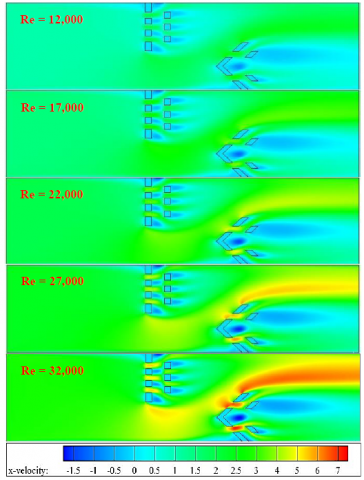Figure 5. u = f (Re)

4.5 Y-velocity fields

The velocity values are also analyzed according to the vertical axis of the exchanger (v) as the speed values are low over the entire structure of the exchanger, except for those areas adjacent to the lower VBs. There is already an improvement in the vertical component of velocity as its greatest on the upper right wing of the FLVB, while it is less valuable near the solid lower part of the FUFB (Figure 6). At the highest flow rate (Re = 32 × 103), the optimum v is 5.18 m.s-1. This speed decreases by 16% by decreasing the flow rate to 27 (× 103). Also, 32% when the flow rate drops to 22 (× 103), 48% when the flow rate reduces to 22% and finally, the vertical component of the flow speed is reduced to 64% if the Re falls below its minimum value, Re = 12 (× 103). Therefore, the v component has a direct relationship with its rate.

## 6.png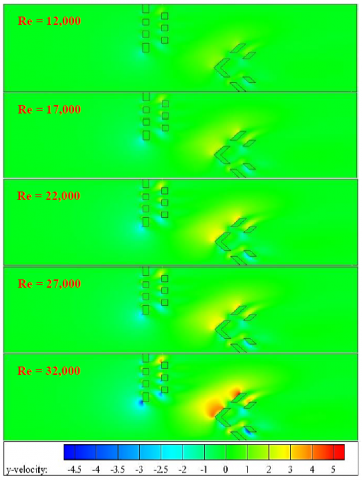Figure 6. v = f (Re)

4.6 Turbulent intensity fields

## 7.pngFigure 7. I = f (Re)

In terms of the intensity (I)of the turbulence, there are zones of low I value as observed from the inlet of the exchanger to the region of the FUFB presence. There are also areas of medium I, that is, those that are centered behind the SUFB and SLVB. In addition, the I values are found in hight intensity in the regions contained the FBs and VBs. Next to the front solid limits of all obstacles, i.e. FUFB, SUFB, FLVB, and SLVB, the disturbance intensity increases significantly. The I across the FBs and VBs holes also improves dramatically in relation to those values across the gaps. In general, the Is are very high in the deflector regions compared to those in the same zones without turbulators and this, mainly highlights the importance of these FBs and VBs in improving the exchanger structure also in terms of the turbulence intensity. The I value is maximum in the case of the best flow rate (Figure 7).

4.7 Turb-viscosity fields

Investigating the viscosity (μt) values and their change for different flow rates is shown in the following figure, which mainly highlights two main zones for high μt values, namely, the centering in front of the attack head of the FLVB, while the rest of the other values are located at the exit of the exchanger, where they are centered. Also, there is a change in the degree of viscosity according to the change in the rate of flow, as they are proportional (Figure 8).

## 8.pngFigure 8. μ = f (Re)

4.8 Turb kinetic-energy and dissipation-rate fields

The study also shows the development of the k flow energy in the presence of two distinct currents, main and secondary, for a continuous flow rate from 12 to 32 (× 103). The plot gives a different structure in the presence of the FBs and VBs compared to those zones that do not contain them (Figure 9). The plot clearly shows that there is an improvement across the deflector holes while there is a failure throughout the exchanger structure. The fluid field reflects the effect of both factors, the deflectors (FBs and VBs) and the flow rate, on energy development, as it is proportional to Reynolds values that is, as it improves, the energy values rise near the baffles. On the other hand, the ε rate changes with the same behavior of the k energy. It is also proportional to the Re rate (Figure 10).

## 9.png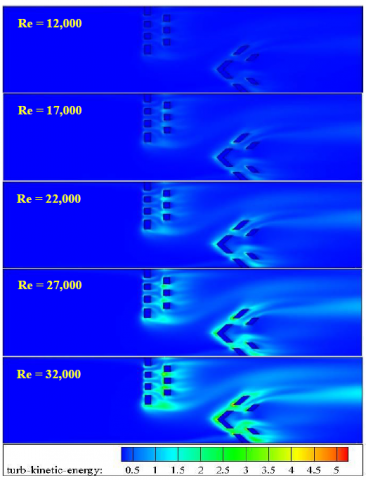Figure 9. k = f (Re)

## 10.png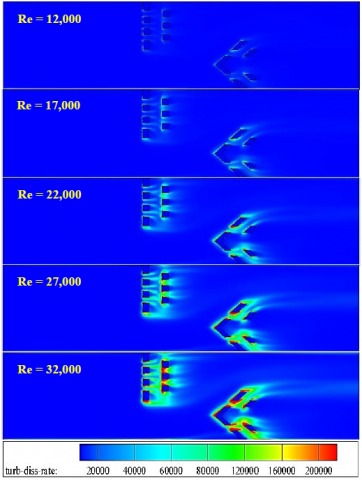Figure 10. ε = f (Re)

4.9 Thermal fields

The heat exchanger has a hot surface with a constant temperature of 375 K. The FUFB is also subjected to the same temperature as the top surface of the exchanger, while the rest of the surfaces are all insulated. This thermal boundary condition has been used by many researchers such as Menni et al. [18-35], and Nasiruddin and Kamran Siddiqui . The fluid T has high values near the hot solid boundaries and low values over the rest of the flow regions (Figure 11). Higher T values are found on both sides of the FUFB, especially on the front. The area containing the hot deflector is higher in terms of temperature compared to the same area in the absence of the fin, as confirmed by many studies [18-35, 37]. On the other hand, high T gradients (TGs) are evident on the front and undersides of the solid parts from the FUFB, and also along the hot exchanger wall behind the SUFB. However, the lower T gradients are centered around the attachment base of the FUFB.

Secondary currents passing through the holes completely eliminate the recirculating cells to avoid low T gradients in the area behind the fins. The effect of the flow rate is also shown in the same figure. The analysis of the heat behavior of the exchanger indicates that the T gradients improve as the flow rate improves. It means that there is a direct proportionality between the two variables (Re and TG).

## 11.png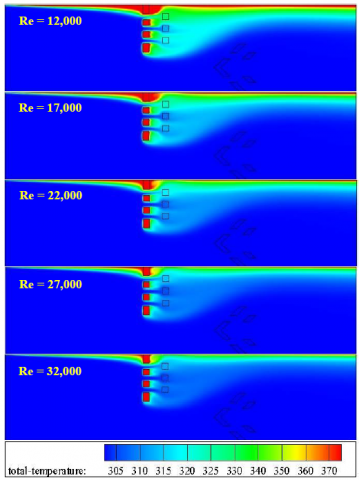Figure 11. T = f (Re)

4.10 Thermal fields

The plots for both Pd and u/Uin at the exchanger’s outlet are illustrated in Figures 12a and 12b, respectively. Their maximum values are 22.65 Pa and 5.8, respectively. There is a reduction of about 85 and 62 percent if the flow rate drops to its minimum value.

## 12a.png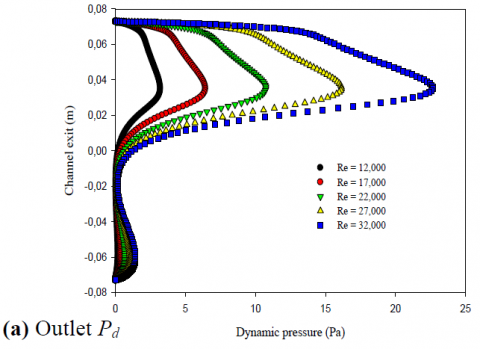## 12b.png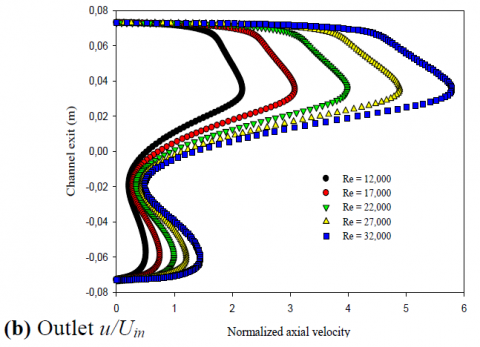## 12c.png## 12d.png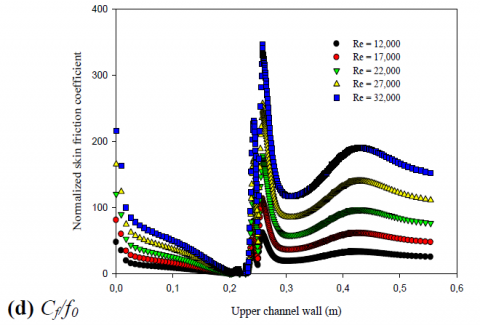Figure 12. Hydrothermal parameters in function of Re

Heat exchange values are greatest in the vicinity of the upper surface of the SUFB (Figures 12c). This region has high T gradients because the flow is subjected to high Pd and V. However, the transfer of the heat decreases near the base of the FUFB in all flow rate cases. Friction values (Cf/f0) are also high in the high T gradient regions, as well as to the right of the lower deflectors (VBs) (Figures 12d). All of these amounts change positively with the flow rate.

5. Conclusion

Details on the hydrothermal characteristics inside a heat exchanger provided with discontinuous FBs and VBs were presented.

1. The role of the secondary flows is to eliminate all the recycling zones behind all the FBs and VBs, so this effectively reflects the presence of the discontinuous deflectors. Also, the flow lines are greatly strained with the continuous increase in flow rates.
2. Secondary streams flow through the holes of both adjacent FUFB and SUFB. While, there is a main flied below these upper deflectors, it also flows in parallel lines until it reaches the FLVB and SLVB.
3. The pressure changes with the evolution of the flow rate from 12 to 32 (×103), being 45 Pa at Remax.
4. Secondary flows through holes have high speeds, due to high Pd over the entire frontal area of these deflectors in both cases simulated.
5. The main currents that traverse the two gaps, the lower, i.e. below the FUFB and SUFB, and the upper, i.e. above the FLVB and SLVB, increase in speed, due to the increased Pd in the region.
6. The V value in the case of Remin is estimated at 3.18 m.s-1, i.e. an average value of 0.95 m.s-1. These values improve to 8.73 m.s-1 as the maximum velocity while to 2.62 m.s-1 as the medium value in the case of the highest flow rate.
7. The u reaches large values in the last side of the exchanger, at its upper part, reaching 2.72 m.s-1 for the lowest flow rate, increasing by 43% with an increase in the Re to 17 (×103), 86% in the case of the next rate (Re = 22 × 103), 129% in the case of Re = 27 (×103), while 172% in the case of the Re maximum (Re = 32 × 103).
8. At the highest flow rate, the optimum v is 5.18 m.s-1. This speed decreases by 16% by decreasing the flow rate to 27 (× 103). Also, 32% when the flow rate drops to 22 (× 103), 48% when the flow rate reduces to 22% and finally, the vertical component of the flow speed is reduced to 64% if the Re falls below its minimum value, Re = 12 (× 103).
9. The area containing the hot deflector is higher in terms of temperature compared to the same area in the absence of the fin.
10. Secondary currents passing through the holes completely eliminate the recirculating cells to avoid low T gradients in the area behind the fins.
11. Heat exchange values are greatest in the vicinity of the upper surface of the SUFB. This region has high T gradients because the flow is subjected to high Pd and V. Friction values are also high in the high T gradient regions, as well as to the right of the lower deflectors (VBs) and, all of these amounts change positively with the flow rate.
References

 Menni, Y., Chamkha, A.J., Azzi, A. (2019). Nanofluid flow in complex geometries - a review. Journal of Nanofluids, 8(5): 893-916. https://doi.org/10.1166/jon.2019.1663

 Menni, Y., Chamkha, A.J., Lorenzini, G., Kaid, N., Ameur, H., Bensafi, M. (2019). Advances of nanofluids in solar collectors - a review of numerical studies. Mathematical Modelling of Engineering Problems, 6(3): 415-427. https://doi.org/10.18280/mmep.060313

 Menni, Y., Chamkha, A.J., Ameur, H. (2020). Advances of nanofluids in heat exchangers - a review. Heat Transfer, 49(8): 4321-4349. https://doi.org/10.1002/htj.21829

 Menni, Y., Chamkha, A.J., Kaid, N., Ameur, H., Bensafi, M., Sahel, D., Lorenzini, G. (2020). Advances of heat transfer in porous media - a review. Special Topics & Reviews in Porous Media, 11(1): 1-18. https://doi.org/10.1615/SpecialTopicsRevPorousMedia.2020028581

 Menni, Y., Chamkha, A.J., Azzi, A. (2019). Nanofluid transport in porous media - a review. Special Topics & Reviews in Porous Media, 10(1): 49-64. https://doi.org/10.1615/SpecialTopicsRevPorousMedia.2018027168

 Menni, Y., Azzi, Y., Chamkha, A.J. (2018). A review of solar energy collectors: Models and applications. Journal of Applied and Computational Mechanics, 4(4): 375-401. https://doi.org/10.22055/JACM.2018.25686.1286

 Menni, Y., Azzi, A., Chamkha, A.J. (2019). Enhancement of convective heat transfer in smooth air channels with wall-mounted obstacles in the flow path. Journal of Thermal Analysis and Calorimetry, 135(4): 1951-1976. https://doi.org/10.1007/s10973-018-7268-x

 Menni, Y., Ameur, H., Inc, M. (2020). Improvement of the performance of solar channels by using vortex generators and hydrogen fluid. Journal of Thermal Analysis and Calorimetry, 1-22. https://doi.org/10.1007/s10973-020-10239-3

 Menni, Y., Chamkha, A.J., Ghazvini, M., Ahmadi, M.H., Ameur, H., Issakhov, A., Inc, M. (2020). Enhancement of the turbulent convective heat transfer in channels through the baffling technique and oil/multiwalled carbon nanotube nanofluids. Numerical Heat Transfer, Part A: Applications, 1-41. https://doi.org/10.1080/10407782.2020.1842846

 Santos, N.B., de Lemos, M.J.S. (2006). Flow and heat transfer in a parallel-plate channel with porous and solid baffles. Numerical Heat Transfer, Part A, 49: 471-494. https://doi.org/10.1080/10407780500325001

 Dutta, P., Hossain, A. (2005). Internal cooling augmentation in rectangular channel using two inclined baffles. International Journal of Heat and Fluid Flow, 26: 223-232. https://doi.org/10.1016/j.ijheatfluidflow.2004.08.001

 Kamali, R., Binesh, A.R. (2008). The importance of rib shape effects on the local heat transfer and flow friction characteristics of square ducts with ribbed internal surfaces. International Communications in Heat and Mass Transfer, 35: 1032-1040. https://doi.org/10.1016/j.icheatmasstransfer.2008.04.012

 Wang, F., Zhang, J., Wang, S. (2012). Investigation on flow and heat transfer characteristics in rectangular channel with drop-shaped pin fins. Propulsion and Power Research, 1(1): 64-70. https://doi.org/10.1016/j.jppr.2012.10.003

 Fawaz, H.E., Badawy, M.T.S., Abd Rabbo, M.F., Elfeky, A. (2017). Numerical investigation of fully developed periodic turbulent flow in a square channel fitted with 45° in-line V-baffle turbulators pointing upstream. Alexandria Engineering Journal, 57(2): 633-642. http://dx.doi.org/10.1016/j.aej.2017.02.020

 Boonloi, A., Jedsadaratanachai, W. (2016). Numerical investigation on turbulent forced convection and heat transfer characteristic in a square channel with discrete combined V-baffle and V-orifice. Case Studies in Thermal Engineering, 8(2016) 226-235. https://doi.org/10.1016/j.csite.2016.07.003

 Tamna, S., Skullong, S., Thianpong, C., Promvonge, P. (2014). Heat transfer behaviors in a solar air heater channel with multiple V-baffle vortex generators. Solar Energy, 110: 720-735. https://doi.org/10.1016/j.solener.2014.10.020

 Nanan, K., Thianpong, C., Pimsarn, M., Chuwattanakul, V., Eiamsaard, S. (2016). Flow and thermal mechanisms in a heat exchanger tube inserted with twisted cross-baffle turbulators. Applied Thermal Engineering, 114: 130-147. https://doi.org/10.1016/j.applthermaleng.2016.11.153

 Menni, Y., Chamkha, A., Zidani, C., Benyoucef, B. (2020). Baffle orientation and geometry effects on turbulent heat transfer of a constant property incompressible fluid flow inside a rectangular channel. International Journal of Numerical Methods for Heat & Fluid Flow, 30(6): 3027-3052. https://doi.org/10.1108/HFF-12-2018-0718

 Menni, Y., Azzi, A., Chamkha, A.J., Harmand, S. (2019). Effect of wall-mounted V-baffle position in a turbulent flow through a channel Analysis of best configuration for optimal heat transfer. International Journal of Numerical Methods for Heat & Fluid Flow, 29(10): 3908-3937. https://doi.org/10.1108/HFF-06-2018-0270

 Menni, Y., Azzi, A., Chamkha, A. (2019). Modeling and analysis of solar air channels with attachments of different shapes. International Journal of Numerical Methods for Heat & Fluid Flow, 29(5): 1815-1845. https://doi.org/10.1108/HFF-08-2018-0435

 Menni, Y., Ameur, H., Yao, S.W., Amraoui, M.A., Inc, M., Lorenzini, G., Ahmad, H. (2021). Computational fluid dynamic simulations and heat transfer characteristic comparisons of various arc-baffled channels. Open Physics, 19: 51-60. https://doi.org/10.1515/phys-2021-0005

 Menni, Y., Chamkha, A.J., Massarotti, N., Ameur, H., Kaid, N. (2020). Hydrodynamic and thermal analysis of water, ethylene glycol and water-ethylene glycol as base fluids dispersed by aluminum oxide nano-sized solid particles. International Journal of Numerical Methods for Heat & Fluid Flow, 30(9): 4349-4386. https://doi.org/10.1108/HFF-10-2019-0739

 Menni, Y., Ameur, H., Chamkha, A.J., Inc, M., Almohsen, B. (2020). Heat and mass transfer of oils in baffled and finned ducts. Thermal Science, 24(1): 267-276. https://doi.org/10.2298/TSCI20S1267M

 Menni, Y., Ghazvini, M., Ameur, H., Ahmadi, M.H., Sharifpur, M., Sadeghzadeh, M. (2020). Numerical calculations of the thermal-aerodynamic characteristics in a solar duct with multiple V-baffles. Engineering Applications of Computational Fluid Mechanics, 14(1): 1173-1197. https://doi.org/10.1080/19942060.2020.1815586

 Menni, Y., Ameur, H., Sharifpur, M., Ahmadi, M.H. (2021). Effects of in-line deflectors on the overall performance of a channel heat exchanger. Engineering Applications of Computational Fluid Mechanics. https://doi.org/10.1080/19942060.2021.1893820

 Menni, Y., Azzi, A., Chamkha, A.J. (2019). Computational thermal analysis of turbulent forced-convection flow in an air channel with a flat rectangular fin and downstream v-shaped baffle. Heat Transfer Research, 50(18): 1781-1818. https://doi.org/10.1615/HeatTransRes.2019026143

 Menni, Y., Azzi, A., Chamkha, A.J., Harmand, S. (2019). Analysis of fluid dynamics and heat transfer in a rectangular duct with staggered baffles. Journal of Applied and Computational Mechanics, 5(2): 231-248. https://doi.org/10.22055/JACM.2018.26023.1305

 Menni, Y., Azzi, A., Chamkha, A.J. (2019). The solar air channels: comparative analysis, introduction of arc-shaped fins to improve the thermal transfer. Journal of Applied and Computational Mechanics, 5(4): 616-626. https://doi.org/10.22055/JACM.2018.26785.1356

 Menni, Y., Chamkha, A.J., Azzi, A. (2020). Fluid flow and heat transfer over staggered ꞌ+ꞌ shaped obstacles. Journal of Applied and Computational Mechanics, 6(4): 741-756. https://doi.org/10.22055/JACM.2018.26277.1316

 Menni, Y., Azzi, A., Zidani, C. (2017). Use of waisted triangular-shaped baffles to enhance heat transfer in a constant temperature-surfaced rectangular channel. Journal of Engineering Science and Technology, 12(12): 3251-3273.

 Menni, Y., Azzi, A., Chamkha, A.J. (2018). Turbulent heat transfer and fluid flow over complex geometry fins. Defect and Diffusion Forum, 388: 378-393. https://doi.org/10.4028/www.scientific.net/DDF.388.378

 Menni, Y., Ameur, H., Amghar, K., Maouedj, R. (2021). The importance of the finning technology in modernizing simple solar air-heat exchangers. Materials Today: Proceedings. https://doi.org/10.1016/j.matpr.2021.02.363

 Menni, Y., Azzi, A. (2018). Numerical analysis of thermal and aerodynamic fields in a channel with cascaded baffles. Periodica Polytechnica Mechanical Engineering, 62(1): 16-25. https://doi.org/10.3311/PPme.10613

 Menni, Y., Azzi, A., Zidani, C., Benyoucef, B. (2016). Numerical analysis of turbulent forced-convection flow in a channel with staggered l-shaped baffles. Journal of New Technology and Materials, 6(2): 44-55. https://doi.org/10.12816/0043933

 Menni, Y., Chamkha, A.J., Zidani, C., Benyoucef, B. (2019). Heat transfer in air flow past a bottom channel wall-attached diamond-shaped baffle - using a CFD technique. Periodica Polytechnica Mechanical Engineering, 63(2): 100-112. https://doi.org/10.3311/PPme.12490

 Demartini, L.C., Vielmo, H.A., Möller, S.V. (2004). Numeric and experimental analysis of the turbulent flow through a channel with baffle plates. J. Braz. Soc. Mech. Sci. Eng., 26(2): 153-159. https://doi.org/10.1590/S1678-58782004000200006

 Nasiruddin, M.H., Siddiqui, K. (2007). Heat transfer augmentation in a heat exchanger tube using a baffle. International Journal of Heat and Fluid Flow, 28(2): 318-328. https://doi.org/10.1016/j.ijheatfluidflow.2006.03.020

 Derakhshan, M.M., Akhavan-Behabadi, M.A. (2016). Mixed convection of MWCNT-heat transfer oil nanofluid inside inclined plain and microfin tubes under laminar assisted flow. International Journal of Thermal Sciences, 99: 1-8. https://doi.org/10.1016/j.ijthermalsci.2015.07.025

 Gholami, M.R., Akbari, O.A., Marzban, A., Toghraie, D., Shabani, G.A.S., Zarringhalam, M. (2018). The effect of rib shape on the behavior of laminar flow of oil/MWCNT nanofluid in a rectangular microchannel. Journal of Thermal Analysis and Calorimetry, 134(3): 1611-1628. https://doi.org/10.1007/s10973-017-6902-3

 Launder, B.E., Spalding, D.B. (1974). The numerical computation of turbulent flows. Computer Methods in Applied Mechanics and Engineering, 3: 269-289. https://doi.org/10.1016/0045-7825(74)90029-2

 Yang, Y.T., Hwang, C.Z. (2003). Calculation of turbulent flow and heat transfer in a porous-baffled channel. International Journal of Heat and Mass Transfer, 46(5): 771-780. https://doi.org/10.1016/S0017-9310(02)00360-5

 Biçer, N., Engin, T., Yasar, H., Büyükkaya, E., Aydın, A., Topuz, A. (2020). Design optimization of a shell-and-tube heat exchanger with novel three-zonal baffle by using CFD and Taguchi method. International Journal of Thermal Sciences, 155: 106417. https://doi.org/10.1016/j.ijthermalsci.2020.106417

 Lv, W., Ou, G., Liu, X., Gong, C. (2020). Experimental and numerical studies on the corrosion properties of AISI 316L stainless steel in two-phase upward slug flows. Engineering Applications of Computational Fluid Mechanics, 14(1): 897-909. https://doi.org/10.1080/19942060.2020.1780155

 Maouedj, R., Menni, Y., Inc, M., Chu, Y.M., Ameur, H., Lorenzini, G. (2021). Simulating the turbulent hydrothermal behavior of Oil/MWCNT nanofluid in a solar channel heat exchanger equipped with vortex generators. Computer Modeling in Engineering & Sciences, 126(3): 855-889. https://doi.org/10.32604/cmes.2021.014524

 Patankar, S.V. (1980). Numerical Heat Transfer and Fluid Flow. McGraw-Hill, New York, NY.

 Leonard, B.P., Mokhtari, S. (1990). Ultra-sharp nonoscillatory convection schemes for high-speed steady multidimensional flow. NASA TM1-2568, NASA Lewis Research Center, 1990.